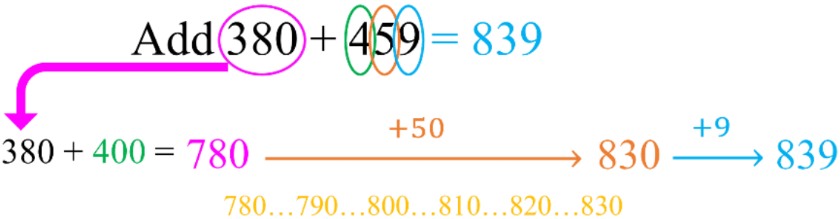#### All in One Place

Everything you need for better grades in university, high school and elementary.#### Learn with Ease

Made in Canada with help for all provincial curriculums, so you can study in confidence.#### Instant and Unlimited Help

0/5
##### Intros
###### Lessons
2. Mental math and sums of 10
0/21
##### Examples
###### Lessons
1. Adding by grouping sums of 10
Add by pairing up sums of 10. Circle the pair and fill in the blanks.
1. 8 + 5 + 2 = ____
10 + ____ = ____
2. 1 + 7 + 3 = ____
10 + ____ = ____
3. 6 + 9 + 4 = ____
10 + ____ = ____
Add 9 by: adding 10 first, then taking 1 away. Fill in the blanks.
1. 7 + 9 = ____
7 + 10 = ____ - 1 = ____
2. 14 + 9 = ____
14 + 10 = ____ - 1 = ____
3. 38 + 9 = ____
38 + 10 = ____ - 1 = ____
Add 8 by: adding 10 first, then taking 2 away. Fill in the blanks.
1. 7 + 8 = ____
7 + 10 = ____ - 2 = ____
2. 16 + 8 = ____
16 + 10 = ____ - 2 = ____
3. 54 + 8 = ____
54 + 10 = ____ - 2 = ____
Add by changing one number into a "friendly" number and adjust. Fill in the blanks.
1.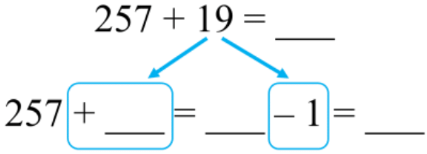2.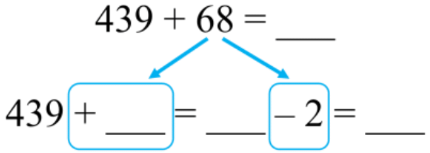3.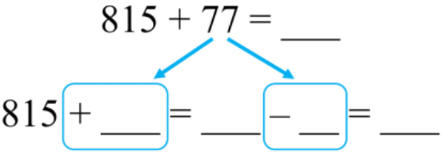1.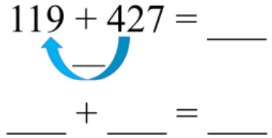2.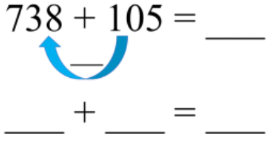3.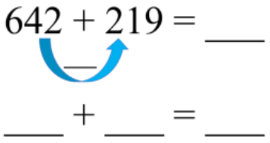Use the method of adding on from left to right.
1. 553 + 385 = ____
2. 730 + 291 = _____
3. 664 + 148 = ____
7. Counting on from the first number
Add by counting on from the first number
1. 104 + 693 = ____
2. 827 + 335 = _____
3. 568 + 429 = ____
0%
##### Practice
###### Topic Notes

In this lesson, we will learn:

• The addition strategies of: (1) using friendly numbers with adjustment, (2) creating friendly numbers using addends, (3) adding on from left to right, and (4) adding by counting on

Notes:

• Method 1: change into a "friendly" number and then adjust (by subtracting)
• Round one of the addends to the nearest multiple of 10
• Then adjust by taking away how much you rounded up by to get the answer• Method 2: make a "friendly" number using the addends
• Use the numbers that were given for the addition equation
• Move values from one number to the other number to make a multiple of 10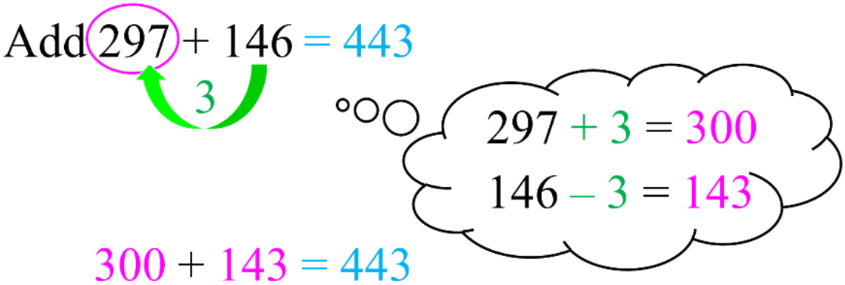• Method 3: adding from left to right
• Add by going through one place value at a time
• i.e. hundreds of 1st # + hundreds 2nd # + tens of 1st # + tens of 2nd # + . . .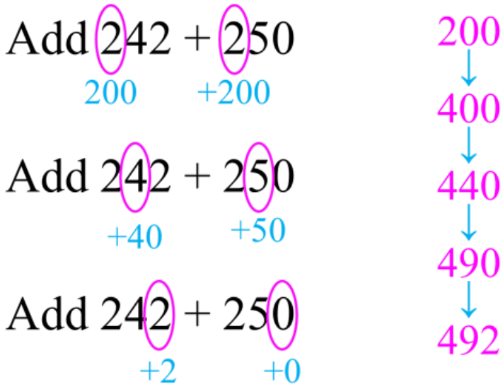• Method 4: adding by counting on
• Start with the first number, then add the second number one place value at a time